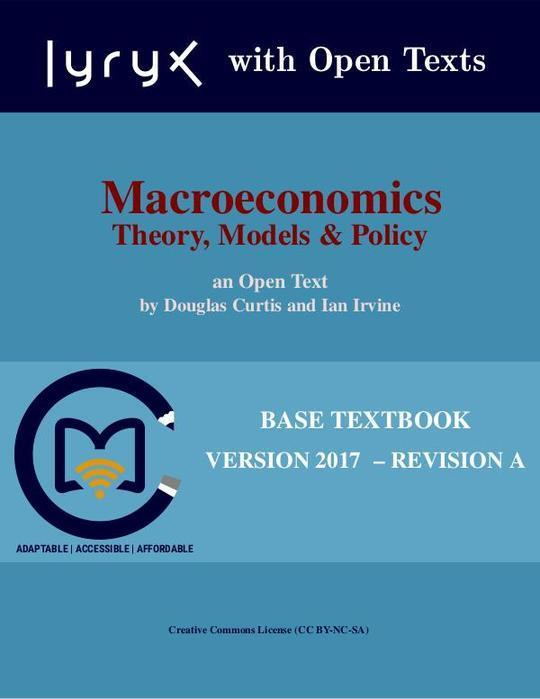Free

# CurtisIrvine-Macroeconomics-2017A

By Unknown
Free
The publisher has enabled DRM protection, which means that you need to use the BookFusion iOS, Android or Web app to read this eBook. This eBook cannot be used outside of the BookFusion platform.
Book Description
• Part One: Introduction
• Introduction to key ideas
• Understanding through the use of models
• Opportunity cost and the market
• A model of exchange and specialization
• Economy-wide production possibilities
• Aggregate output, growth and business cycles
• Key concepts
• End of chapter exercises
• Theories, models and data
• Observations, theories and models
• Variables, data and index numbers
• Testing economic models & analysis
• Diagrams and economic analysis
• Ethics, efficiency and beliefs
• Key concepts
• End of chapter exercises
• The classical marketplace – demand and supply
• The market's building blocks
• Demand and supply curves
• Other influences on demand
• Other influences on supply
• Simultaneous supply and demand impacts
• Market interventions
• Individual and market functions
• Key concepts
• End of chapter exercises
• Part Two: Introduction to Macroeconomics
• Economic activity and performance
• Macroeconomic performance
• National accounts
• Measuring GDP
• Nominal GDP, real GDP & the GDP deflator
• Per capita real GDP
• Key concepts
• End of chapter exercises
• Output, business cycles, growth & employment
• Aggregate demand & aggregate supply
• Equilibrium output and potential output
• Growth in potential output
• Business cycles and output gaps
• Output gaps and unemployment rates
• The role of macroeconomic policy
• Key concepts
• End of chapter exercises
• Aggregate expenditure & aggregate demand
• Short run aggregate demand and output
• Consumption, saving, and investment
• The export and import functions
• Aggregate expenditure and equilibrium output in the short run
• The multiplier: changes in aggregate expenditure and equilibrium output
• Equilibrium output and the AD curve
• Key concepts
• End of chapter exercises
• The government sector
• Government expenditure, taxes, and equilibrium real GDP
• The government's budget and budget balance
• Fiscal policy & government budget balances
• Automatic and discretionary fiscal policy
• The public debt and the budget balance
• Aggregate demand & equilibrium output
• Key concepts
• End of chapter exercises
• Part Three: Financial Markets & Economic Activity
• Money, banking & money supply
• Money and the functions of money
• Measures of the Canadian money supply
• Money created by banks
• The monetary base and the money supply
• Key concepts
• End of chapter exercises
• Financial markets, interest rates, foreign exchange rates & AD
• Portfolio choices between money and other assets
• Bond prices, yields and interest rates
• The demand for money balances
• Financial market equilibrium & interest rates
• Interest rates and foreign exchange rates
• Interest rates, exchange rates, and aggregate demand
• The transmission mechanism
• Key concepts
• End of chapter exercises
• Central banking and monetary policy
• Central banking and the Bank of Canada
• Central bank operating techniques
• Monetary policy objectives & instruments targets
• Monetary policy rules
• The long-run neutrality of money
• Monetary policy indicators
• Key concepts
• End of chapter exercises
• Part Four: Real GDP, Business Cycles, Policy and Growth
• The construction of an AD curve
• The slope and position of the AD curve
• Short-run aggregate supply
• Short-run equilibrium output and prices
• Business cycles in output and prices
• Internal adjustment to output gaps?
• Monetary policy and fiscal policy
• Key concepts
• End of chapter exercises
• An AD/ AS model of the inflation rate and real GDP
• Inflation and aggregate demand
• Aggregate supply
• The equilibrium inflation rate
• Monetary and fiscal policies
• Recession, disinflation and deflation
• Key concepts
• End of chapter exercises
• Economic growth
• Growth in potential output
• Growth in per capita GDP
• Technology & growth in per capita output
• Neoclassical growth theory and the convergence hypothesis
• Recent growth studies and policy issues
• Key concepts
• End of chapter exercises
• Part Five: International Macroeconomics and Trade Theory
• International Macroeconomics
• The balance of payments
• The foreign exchange market
• Flexible exchange rates and fixed exchange rates
• Monetary and fiscal policy with flexible exchange rates
• Monetary and fiscal policy with fixed exchange rates
• Key concepts
• End of chapter exercises